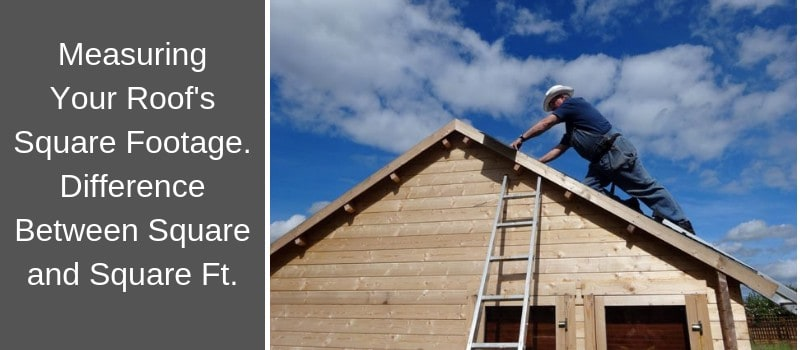## Roof Measuring

### How do I calculate the size of my roof?

Multiply your house length by your house width to get the area. (For example, 40 feet x 30 feet = 1,200 square feet.) Next, multiply the area by your roof’s pitch. (1,200 x 1.05 = 1,260 square feet.)

Can you measure a roof from the ground?

If you’re planning work on a roof and don’t have the building plans, taking preliminary measurements from the ground allows you to approximate the roof area and estimate some costs. … But, you can measure a gable or flat roof from the ground with the help of an assistant.## How to Manually Measure a Roof

To manually measure a roof without the help of aerial measurement reports, you must visit a job site and climb onto the roof to take precise roof measurements by hand.

1. Measure the width and length of each plane of the roof (including dormers), and then multiply those numbers to get the correct figure for the square footage of that area.
2. Note any skylights, chimneys or other parts of the roof that would not require materials so you can subtract those measurements from your total area.
3. Add together the calculated square footage of each surface to get the figure for total square footage of the roof.
4.5. After determining the total square footage of the roof, you must calculate the pitch of the roof by measuring the vertical distance (rise) over a 12 inch horizontal segment (run).
6. Write down these numbers as the vertical measurement listed first and the horizontal measurement recorded second so you can find rise over run.
7. When you have the total square footage and rise over run of the roof, you can calculate the roof squares to determine how much materials are needed to cover the roof. To calculate the number of squares on a roof, you need to divide its total square feet by 100.

## WHAT YOU’LL NEED TO CALCULATE ROOF AREA:

• Large level
• Measuring tape
• Marker
• Pencil, paper### MEASURING THE PITCH

To calculate the area of your roof, first you’ll need to calculate the pitch of it:

1. First, use your measuring tape to measure 12 inches on your large level and make a mark at the 12-inch line.
3. Climb to the top of your roof.
4. After that, place your level against the gable trim and flat against the side of the house.
5. Using your measuring tape, measure from the 12-inch mark on your level up to the bottom edge of your gable trim. This number is your rise.
6. Each rise measurement is written as “# in 12” and this is your roof’s pitch. (For example, if your rise is 4 inches, your roof’s pitch is 4 in 12.)### CALCULATING THE AREA

If you want to get the exact area measurement for your roof, you can follow these steps:

1. Once you have your roof’s pitch, divide the number by 12. (For example, if your roof’s pitch is 4 in 12, you would divide 4 by 12. This would yield 1/3.)
2. Next, square your result. (If your number is 1/3, squared would yield 1/9.)
3. After that, add 1 to your number. (1/9 + 1 = 10/9.)
4. Next, figure out the square root of your new number. (The square root of 10/9 is 1.05.)
5. Next, use your measuring tape to measure the length of your house. (Be sure to include overhangs.)
6. After that, measure the width of your house. (Be sure to include overhangs.)
7. Multiply your house length by your house width to get the area. (For example, 40 feet x 30 feet = 1,200 square feet.)
8. Next, multiply the area by your roof’s pitch. (1,200 x 1.05 = 1,260 square feet.)
9. To allow for hips, ridges, and waste, add 10% of your final number for a gable roof and 17% of your final number for a cottage roof. (Your total number would be either 1,386 or 1,474 square feet.)

When measuring a roof you must ALWAYS draw the layout of the roof. Your roof layout sketch must include the dimensions, slopes, obstacles and penetrations, and any other unusual situations such as areas of soft spots, rotten wood, and anything other obstacles.

Once you have all your measurements you will use them to calculate your areas, slopes, angles, and allowance factors.

Tags: No tags
0